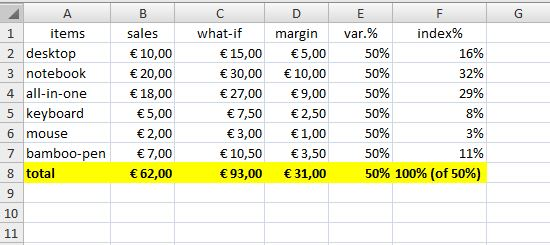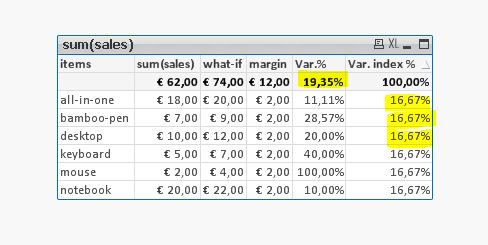# QlikView Creating Analytics

Discussion Board for collaboration related to Creating Analytics for QlikView.

HighlightedNot applicable

## howto calculate index % of margin variation

Dear all,

at the moment I don't understand how to set expression for calculating index % of margin in linear table graph.

In particolar my goal is calculate the distributions % of variation. Enclosed you can find out an example of my goal.

Note: I'm using a bookmark as based selection before calculate sales and what-if.

Any advice or comment is very appreciated.

thank you very much

Simone

Tags (6)
6 Replies
Valued Contributor

## Re: howto calculate index % of margin variation

simone,

Could you post the expected output to better understand your requirement, please?

Marc.Not applicable

## Re: howto calculate index % of margin variation

Hi Marc,

my goal is show in var. index % as described in excel.Cheers

Simone

Valued Contributor

## Re: howto calculate index % of margin variation

ok, then your expression is ok, except of a bracket ) missing at the end.

Marc.Not applicable

## Re: howto calculate index % of margin variation

thank you for your feedback but I don't understand why the variation index % in qlik is always the same and variation is difference. #caosinmindmodeonValued Contributor

## Re: howto calculate index % of margin variation

simone,

I asked and you described the index margin = margin / total margin.  In your sample data your margin is 2€ in all rows, then you will obtain the same index margin. In the excel screenshot you posted before the margin (€) were different, and you got different index margins as well.

Marc.Not applicable

## Re: howto calculate index % of margin variation

I resolved the issue since the incidence % is based on margin(i)/sum(total Sales).

Thank you very much, Marc.

Have a nice day.

S.